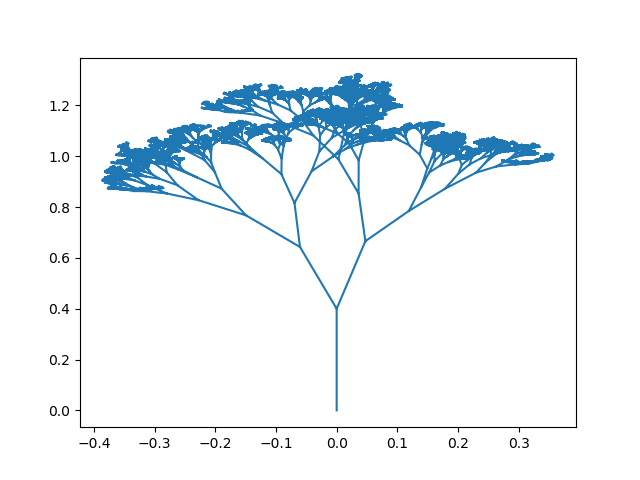# Drawing random trees using geometry and recursionA Tree generated using the program described below

The program used to generate such trees is a simple but beautiful mixture of algorithms’ recursion and simple geometry. The code is available on github and I provide here some explanation of how it works.

The algorithm is a simple recursive approach: From each branch we are going to generate two child branches until we reach some depth d. A branch here is a segment defined by its extremity points.

The child branches will have a different angle from the parent branch. To make the generated tree more realistic it’s better if one branch has an angle smaller than the angle of the parent and the other branch has a higher angle than that of the parent.

We don’t want the angle alpha to be greater than 90° because otherwise we would have something like:

So to have a more realistic tree, it’s better that alpha, which is the maximum deviation of the child branches from the parent branch, is smaller than 45°.

Let’s focus on generating one child branch, once that is done, generation of the second branch follows same calculations.

Given the 2 extremities of the parent branch, we want to generate the 2 extremities of the child branch. The first extremity of the child is already defined as the second extremity of the parent. Of course the first extremity of the child branch can be somewhere between the 2 extremities of the parent, but this was my choice in the program, and it’s easier. So what remains to calculate is only one extremity which is the point (x3, y3) in the figure below:

(x3, y3) can be anywhere in the space, but for a more realistic tree it must verify 2 conditions:

• The first one is discussed earlier and it has to do with the angle of the branch, the deviation should not exceed alpha.
• The second one has to do with the length of the child branch. Generally the parent branch is bigger than the child.

With these two conditions, the red crossed zone is where (x3, y3) should be:

Now we can choose any angle we want in the red zone (which is equivalent to choosing the slope of the branch) and any length between 0 and the parent’s length. The child branch’s angle is chosen randomly between [angle of parent, angle of parent + max deviation]. We compute the angle of the parent from its slope using arctan. Once angle chosen, tan(angle) is the slope of the child.

The length of the child is strictly smaller than the parent length and can be somewhere between [min_ratio*parent_length, max_ratio*parent_length] where min_ratio and max_ratio are in ]0, 1[. In the program I chose min_ratio=0.5 and max_ratio=0.8.

Once angle and length are fixed, we have a unique point (x3, y3), which is the solution of the two equations below:

The first one describes the length of the branch, the second one describes the slope of the branch.

Now we can solve the two equations to get the formulas that calculate (x2, x3)

Here is the function that does this computations:

Now for the second branch we choose a new length and a new angle, but this time the angle should be between [angle of parent-max deviation, angle of parent]. And same calculations are done.

Now comes the part where we display the branches. With python, matplotlib is the classical library for this. It is used mostly for displaying functions. To display a function you give the plot function two arrays xs and ys which contain points that the function passes through, and plot can link between these points using straight lines. Here, it’s a little bit different because we are not drawing a polyline (which means a broken line that can be drawn continuously with the hand), because once we draw the child branch we want to go back to the first extremity of this branch and draw the second child branch. A little trick for this is to insert the value numpy.nan and then insert the new branch extremities.

Here is the main function that draws a subtree starting from a parent branch:

This is why Math is fun ;)

Computer Science, Physics & Maths Enthusiast